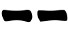## Thursday 2 June 2016

There are many ways of using playing cards to cast a hexagram. I devised the ones described here myself. Frankly I think they are so obvious that probably someone has already described them somewhere else. In case, just let me know and I'll give proper credit.

To cast an hexagam, proceed as follows:
1. Shuffle the deck
2. Pick a card;
3. If the suit is spades (), write down 2; Otherwise, write down 3;
4. Pick another card;
5. If the suit is black, write down 2; Otherwise, write down 3;
6. Pick a third card;
7. If the suit is black, write down 2; Otherwise, write down 3;
8. Sum up the numbers and draw the line according the following table:
 6 7 8 99. Reinsert the three cards in the deck
10. Repeat steps 1-9 other five times drawing the resulting lines from the bottom to the top of the hexagram.
Probabilities
The calculations are simple, just a little bit tedious. The diagrams below summarize all the possible events and their probability:

By multiplying and then summing up the above probabilities we get:
Prob(6) =    5.88% (-0.37%)
Prob(8) =   43.75% (+0.86%)
Prob(7) =  31.25% (+0.12%)
Prob(9) =  18.14% (-0.61%)
Prob(yin) = 50.49%
Prob(yang) = 49.51%

Compared with the yarrow stalks method (see the numbers between parenthesis) this methood is less likely to produce moving lines. Also, it slightly (0.5%) favours yin lines over yang lines.

#### Getting Yarrow Stalks probabilities

If the cards reinserted in the deck the actual probabilities are exactly the same of the yarrow stalks method.
1. Shuffle the deck
2. Pick a card;
3. If the suit is spades (), write down 2; Otherwise, write down 3;
4. Reinsert the card and reshuffle the deck
5. Pick another card;
6. If the suit is black, write down 2; Otherwise, write down 3;
7. Repeat steps 4-6 once again
8. Reinsert the card in the deck
9. Sum up the numbers and draw the line according the following table:
 6 7 8 910. Repeat steps 1-9 other five times drawing the resulting lines from the bottom to the top of the hexagram.
It's easy to see that (having each suit the same number of cards) for the first card the probability to get 2 is 1/4 and the probability to get 3 is 3/4; for the second and third cards the probability to get 2 or 3 is 1/2. This is exactly what happens while counting the traditional 49 yarrow stalks but it is true only if the card is reinserted in the deck at each step.

#### Getting three coins probabilities

The more logical variation to get the three coins method probabilities, is to always assign 2 to black cards and 3 to red cards:

1. Shuffle the deck
2. Pick three cards;
3. For each black suit, count 2; for each red suit, count 3;
4. Sum up the numbers and draw the line according the following table:
 6 7 8 95. Reinsert the three cards in the deck
6. Repeat steps 1-5 other five times drawing the resulting lines from the bottom to the top of the hexagram.
Counting the possible events and their probability, as we did for the first method, gives us the following probabilities.

Prob(6) =  11.76% (-0.74%)
Prob(8) =   38.24% (+0.74%)
Prob(7) =  38.24% (+0.74%)
Prob(9) =  11.76% (-0.74%)
Prob(yin) = Prob(yang) = 50%

Where the numbers in parenthesis represent the discrepancy from the three coins methods. The chance of getting a moving line is lower; the probability of getting  yin or yang is 1/2.

Again, reinserting the card at each step (and reshuffling) would give exactly the three coins method probabilities.

Cards images: AtlasnyeThis work is licensed under a Creative Commons Attribution-NonCommercial 4.0 International License.